# MA.912.GR.1.6Export Print
Solve mathematical and real-world problems involving congruence or similarity in two-dimensional figures.

### Clarifications

Clarification 1: Instruction includes demonstrating that two-dimensional figures are congruent or similar based on given information.
General Information
Subject Area: Mathematics (B.E.S.T.)
Strand: Geometric Reasoning
Status: State Board Approved

## Benchmark Instructional Guide

• Congruent
• Similarity

### Vertical Alignment

Previous Benchmarks

Next Benchmarks

### Purpose and Instructional Strategies

In grade 8 students solved real-world and mathematical problems involving similar triangles, defining similarity in terms of dilations. In Geometry, students extend previous understanding of congruence and similarity to solve mathematical and real-world problems involving congruent or similar polygons. In later courses, students will use similar triangles to develop trigonometry related to the unit circle.
• Instruction includes discussing the definitions of congruent polygons and similar polygons based on corresponding parts. Students should understand that if a problem involves polygons, they will have to use the definitions of congruent and similar (there are no congruence or similarity criteria for polygons) to show the polygons are congruent or similar and use this information to solve the task. (MTR.4.1)
• When two polygons are congruent, corresponding sides and corresponding angles are congruent.
• When two polygons are similar, corresponding angles are congruent and corresponding sides are in proportion.
• Problem types includes using congruence and similarity criteria to determine whether two triangles are congruent or similar, and using the definition of congruence and similarity to find missing angle measures and side lengths.
• Instruction includes the understanding of the geometric mean in right triangles and its connection to similarity criteria.
• Geometric Mean Altitude Theorem
• In a right triangle, the altitude from the right angle to the hypotenuse separates the hypotenuse into two segments. The length of the altitude, in triangle ABC, is the geometric mean between the lengths of the two line segments the altitude creates on the hypotenuse. Therefore, by applying the fact that ΔABCHBAHAC , students can conclude that $\frac{\text{HC}}{\text{HA}}$ = $\frac{\text{HA}}{\text{HB}}$, which is equivalent to HC ⋅ HB = (HA)2 , which is equivalent to $\sqrt{HC · HB}$ = HA.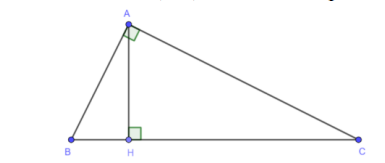• Geometric Mean Leg Theorem
• In a right triangle, the altitude from the right angle to the hypotenuse separates the hypotenuse into two segments. The length of one of the legs, in triangle ABC, is the geometric mean between the length of the hypotenuse and the line segment of the hypotenuse adjacent to that leg. Therefore, by applying the fact  that ΔABCHBAHAC, students can conclude that $\frac{\text{HB}}{\text{BA}}$ = $\frac{\text{BA}}{\text{BC}}$, which is equivalent to HC ⋅ BC = (BA)2, which is equivalent to $\sqrt{HB · BC}$ = BA.• Instruction includes the connection between triangle similarity and the Triangle Proportionality Theorem, or Side-Splitter Theorem. Students can explore and conclude that if a line is parallel to one side of a triangle intersecting the other two sides of the triangle, then the line divides these two sides proportionally.
• For example, given triangle ABC and PQ || AB, students can begin the proof  that $\frac{\text{PA}}{\text{CP}}$ = $\frac{\text{QB}}{\text{CQ}}$ by first proving ΔABCPQC using the Angle-Angle (AA) criterion. Since corresponding sides of similar triangles are in proportion, students can determine the relationship $\frac{\text{CA}}{\text{CP}}$=$\frac{\text{CB}}{\text{CQ}}$. Students should be able to realize that CA = CP + PA and CB = CQ + QB using the segment addition postulate. Therefore, $\frac{\text{CA}}{\text{CP}}$ = $\frac{\text{CB}}{\text{CQ}}$ can be written as $\frac{\text{CP+PA}}{\text{CP}}$ = $\frac{\text{CQ+QB}}{\text{CQ}}$. Students can use their algebraic reasoning to rewrite this relationship equivalently as $\frac{\text{CP}}{\text{CP}}$+$\frac{\text{PA}}{\text{CP}}$ = $\frac{\text{CQ}}{\text{CQ}}$+$\frac{\text{QB}}{\text{CQ}}$ which is equivalent to 1+ $\frac{\text{PA}}{\text{CP}}$ = 1+ $\frac{\text{QB}}{\text{CQ}}$, which is equivalent to $\frac{\text{PA}}{\text{CP}}$ = $\frac{\text{QB}}{\text{CQ}}$.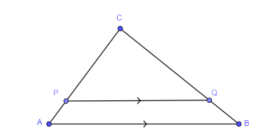### Common Misconceptions or Errors

• Students may have difficulty separating overlapping similar or congruent triangles. To help address this misconception, have students draw the two triangles separately with their corresponding known measures and lengths.
• Students may expect that there are congruence or similarity criteria for non-triangular polygons that are like the criteria for triangles. To help address this misconception, have students create examples in which two quadrilaterals are not congruent even though they have corresponding congruent sides to see that there is no Side-Side-Side-Side congruence criterion for quadrilaterals.

• An artist rendering for the Hapbee Honey Company logo is on a 24” x 36” canvas. The company wants to use the logo on a postcard and is determining the size of the logo based on the different mailing costs. According to the United States Postal Service, mailing costs are determined using the following information.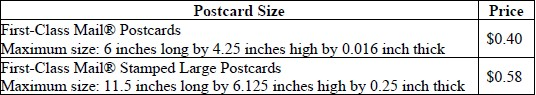• Part A. What is the maximum length of the postcard if it is similar to the original rendering and falls within the First-Class Mail® Postcards dimensions?
• Part B. What is the maximum width of the postcard if it is similar to the original rendering and falls within the First-Class Mail® Stamped Large Postcards dimensions?
• Polygons ABCDE and A'B'C'D'E' are similar and shown.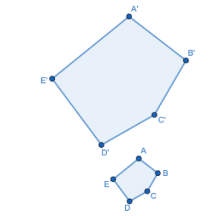• Part A. If $m$A′ = 103°, what is the measure of angle A
• Part B. If $m$D = 97°, what other angle has a measure of 97°?
• Part C. Find the value of $x$ if DC = 2$x$ + 1.5, DC′ = 5.1 and $\frac{\text{BC}}{\text{B'C'}}$ = $\frac{\text{1}}{\text{3}}$
• Figure ABCDEFG is similar to Figure LKJIHFM with a scale factor of 0.5. Assume that the measure of angle B within Figure ABCDEFG is 90°.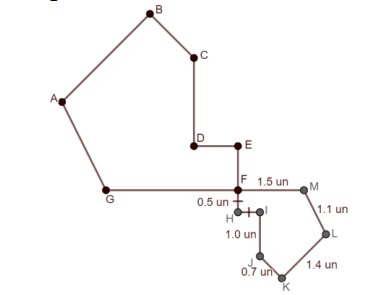• Part A. If point F is located at the origin and line segments EF and CD are vertical and line segments GF and DE are horizontal, determine possible coordinates of each of the points, except points A and B, on Figure ABCDEFG.
• Part B. What is the perimeter of Figure ABCDEFG
• Part C. What is the length of segment DE
• Part D. What is the perimeter of triangle AGC?

### Instructional Items

Instructional Item 1
• Triangles ABC and DEF are shown where ∠A ≅ ∠D, ∠C ≅ ∠F and AC ≅ DF
• Part A. Determine whether the triangles are congruent.
• Part B. If the triangles are congruent, find EF, in units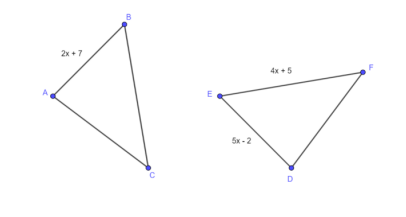Instructional Item 2
• If ΔADE and ΔABC are similar, what is the length of AC, in units?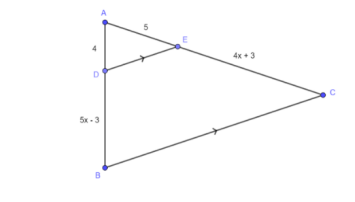*The strategies, tasks and items included in the B1G-M are examples and should not be considered comprehensive.

## Related Courses

This benchmark is part of these courses.
1200400: Foundational Skills in Mathematics 9-12 (Specifically in versions: 2014 - 2015, 2015 - 2022, 2022 and beyond (current))
1206310: Geometry (Specifically in versions: 2014 - 2015, 2015 - 2022, 2022 and beyond (current))
1206320: Geometry Honors (Specifically in versions: 2014 - 2015, 2015 - 2022, 2022 and beyond (current))
7912070: Access Mathematics for Liberal Arts (Specifically in versions: 2014 - 2015, 2015 - 2018, 2018 - 2019, 2019 - 2022, 2022 - 2023, 2023 and beyond (current))
1206315: Geometry for Credit Recovery (Specifically in versions: 2014 - 2015, 2015 - 2022, 2022 and beyond (current))
7912065: Access Geometry (Specifically in versions: 2015 - 2022, 2022 and beyond (current))
1207350: Mathematics for College Liberal Arts (Specifically in versions: 2022 and beyond (current))

## Related Access Points

Alternate version of this benchmark for students with significant cognitive disabilities.
MA.912.GR.1.AP.6: Use the definitions of congruent or similar figures to solve mathematical and/or real-world problems involving two-dimensional figures.

## Related Resources

Vetted resources educators can use to teach the concepts and skills in this benchmark.

## Formative Assessments

Proving Congruence:

Students are asked to explain congruence in terms of rigid motions.

Type: Formative Assessment

County Fair:

Students are given a diagram of a county fair and are asked to use similar triangles to determine distances from one location of the fair to another.

Type: Formative Assessment

Students are asked to decide if a basketball goal is regulation height and are given enough information to determine this using similar triangles.

Type: Formative Assessment

Similar Triangles - 2:

Students are asked to locate a pair of similar triangles in a diagram, explain why they are similar, and use the similarity to find an unknown length in the diagram.

Type: Formative Assessment

## Perspectives Video: Teaching Idea

Measuring Height with Triangles and Mirrors:

Reflect for a moment on how to measure tall objects with mirrors and mathematics.

Type: Perspectives Video: Teaching Idea

## MFAS Formative Assessments

Students are asked to decide if a basketball goal is regulation height and are given enough information to determine this using similar triangles.

County Fair:

Students are given a diagram of a county fair and are asked to use similar triangles to determine distances from one location of the fair to another.

Proving Congruence:

Students are asked to explain congruence in terms of rigid motions.

Similar Triangles - 2:

Students are asked to locate a pair of similar triangles in a diagram, explain why they are similar, and use the similarity to find an unknown length in the diagram.

## Student Resources

Vetted resources students can use to learn the concepts and skills in this benchmark.

## Parent Resources

Vetted resources caregivers can use to help students learn the concepts and skills in this benchmark.